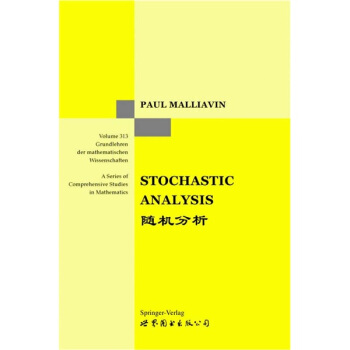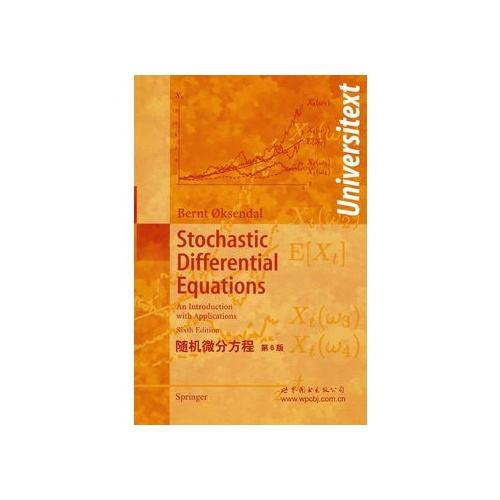## 欢迎访问我的个人主页！

#### 个人简介许明宇，女，‎1978‎年‎1‎月‎26‎日出生，副高级。

• 2000年毕业于山东大学，获学士学位

• 2005年获山东大学理学博士学位与法国Universite du Maine博士学位

• 2006-2008年，复旦大学博士后研究

• 2008年，中国科学院数学与系统科学研究院 助理研究员

• 现任中国科学院数学与系统科学研究院 副研究员

#### 研究方向#### 学术论文

1. Superhedging under ratio constraint.

We consider superhedging of contingent claims under ratio constraint. It has been widely recognized that the minimum cost of superhedging a contingent claim with certain portfolio constraints is equal to the price of a claim with appropriately modified payoff but without constraints. In terms of the backward stochastic differential equation (BSDE) and the variational inequality equation approach, we revisit this result and provide two counterexamples.

2. Numerical algorithms and simulations for reflected backward stochastic differential equations with two continuous barriers.

In this paper we study different algorithms for reflected backward stochastic differential equations (BSDE in short) with two continuous barriers based on the framework of using a binomial tree to simulate 1-d Brownian motion. We introduce numerical algorithms by the penalization method and the reflected method, respectively. In the end simulation results are also presented.

3. The time fractional heat conduction equation in the general orthogonal curvilinear coordinate and the cylindrical coordinate systems.

In this paper a time fractional Fourier law is obtained from fractional calculus. According to the fractional Fourier law, a fractional heat conduction equation with a time fractional derivative in the general orthogonal curvilinear coordinate system is built. The fractional heat conduction equations in other orthogonal coordinate systems are readily obtainable as special cases. In addition, we obtain the solution of the fractional heat conduction equation in the cylindrical coordinate system in terms of the generalized H-function using integral transformation methods. The fractional heat conduction equation in the case 0<α≤1 interpolates the standard heat conduction equation (α=1) and the Localized heat conduction equation (α→0). Finally, numerical results are presented graphically for various values of order of fractional derivative.

4. The fractional diffusion model with an absorption term and modified Fick's law for non-local transport processes.

A generalized non-local Fick’s law on fractal-dimension is derived. Using modified Fick’s law a time–space fractional diffusion model with a fractional oscillator term is built. The solution is obtained in terms of Mittag–Leffler function using finite Hankel integral transformation and Laplace transformation. In addition, numerical simulations are discussed. The results show that the effect range of time-fractional derivative ν on probability density is greater than that of fractional oscillator parameter β. The effect range of ν on probability density is opposite to that of β. This paper provides a new analytical tool to develop fluid mechanics, heat conduction and other engineering science.

5. Axial Couette flow of two kinds of fractional viscoelastic fluids in an annulus.

This paper deals with the unsteady axial Couette flow of fractional second grade fluid (FSGF) and fractional Maxwell fluid (FMF) between two infinitely long concentric circular cylinders. With the help of integral transforms (Laplace transform and Weber transform), generalized Mittag–Leffler function and H-Fox function, we get the analytical solutions of the models. Then we discuss the exact solutions and find some results which have been known as special cases of our solutions. Finally, we analyze the effects of the fractional derivative on the models by using the numerical results and find that the oscillation exists in the velocity field of FMF.

6. Some exact solutions to Stefan problems with fractional differential equations.

Some exact solutions to the first, second and extended Stefan problems with fractional time derivative described in the Caputo sense are given by means of fractional Green's function and Wright function in this paper. By the aid of simple calculations, many results of differential equations of integer order can be obtained as special cases of the results given by this paper.

7. Comparison theorems for forward backward SDEs.

We prove some comparison theorems for forward backward SDEs in 1-dimension or multi-dimension by probabilistic method and duality technique.

8. Backward stochastic differential equations with reflection and weak assumptions on the coefficients.

In this paper, we study reflected BSDE’s with one continuous barrier, under monotonicity and general increasing conditions in y and non-Lipschitz conditions in z. We prove the existence and uniqueness of a solution by an approximation method.

9. Convergence of solutions of discrete reflected backward SDE's and simulations.

The objective of this paper is to introduce elementary discrete reflected backward equations and to give a simple method to discretize in time a (continuous) reflected backward equation. A presentation of numerical simulations is also described.

10. Reflected BSDEs with two barriers under monotonicity and general increasing growth conditions.

In this paper, we prove the existence and uniqueness result of the reflected BSDE with two continuous barriers under monotonicity and general increasing condition on y, with Lipschitz condition on z.

11. g-Gamma Expectations and the Related Nonlinear Doob-Meyer Decomposition Theorem.

In this paper, we define a nonlinear expectation via the BSDE with constraint (BSDE with sigular coefficient), then we introduce definitions of super and sub martingale under this nonlinear expectation and prove their nonlinear Doob-Meyer type decomposition.

12. Reflected Backward Stochastic Differential Equations with two RCLL barriers.

In this paper we consider BSDEs with Lipschitz coefficient reflected on two discontinuous (RCLL) barriers. In this case, we prove first the existence and uniqueness of the solution, then we also prove the convergence of the solutions of the penalized equations to the solution of the RBSDE. Since the method used in the case of continuous barriers (see Cvitanic and Karatzas, Ann. Probab. 24 (1996) 2024–2056 and Lepeltier and San Martín, J. Appl. Probab. 41 (2004) 162–175) does not work, we develop a new method, by considering the solutions of the penalized equations as the solutions of special RBSDEs and using some results of Peng and Xu in Annales of I.H.P. 41 (2005) 605–630.

13. Reflected BSDEs under monotonicity and general increasing growth conditions.

We prove the existence and uniqueness of the solution to certain reflected backward stochastic differential equations (RBSDEs) with one continuous barrier and deterministic terminal time, under monotonicity, and general increasing growth conditions on the associated coefficient. As an application, we obtain, in some constraint cases, the price of an American contingent claim as the unique solution of such an RBSDE.

14. The smallest g-supermartingale and reflected BSDE with single and double L^2 obstacles.

In this paper we show how a solution of BSDE can be reflected by a very irregular L^2-obstacle. We prove that this problem is equivalent to find the smallest g-supermartingale of BSDE that dominates this obstacle. We then obtain the existence and uniqueness and continuous dependence theorem for this reflected BSDE. We also consider the problem of existence and uniqueness of reflected BSDE with double L^2 obstacles, by using a penalization method. A new monotonic limit theorem is developed to prove the convergence of the penalization sequence, and to prove the existence theorem. We also prove that this reflected BSDE with double obstacles is equivalent to a problem of the smallest g-supermartingale and the largest g-submartingale.

15. Penalization method for Reflected Backward Stochastic Differential Equations with on RCLL barrier.

In this paper, we consider BSDEs with a Lipschitz coefficient reflected on one discontinuous (r.c.l.l.) barrier. We prove the convergence of the solutions of the penalized equations to the solution of the RBSDE.

010-62651340

xumy@amss.ac.cn